## Section Exercises

1. When examining the formula of a function that is the result of multiple transformations, how can you tell a horizontal shift from a vertical shift?

2. When examining the formula of a function that is the result of multiple transformations, how can you tell a horizontal stretch from a vertical stretch?

3. When examining the formula of a function that is the result of multiple transformations, how can you tell a horizontal compression from a vertical compression?

4. When examining the formula of a function that is the result of multiple transformations, how can you tell a reflection with respect to the x-axis from a reflection with respect to the y-axis?

5. How can you determine whether a function is odd or even from the formula of the function?

6. Write a formula for the function obtained when the graph of $f\left(x\right)=\sqrt{x}$ is shifted up 1 unit and to the left 2 units.

7. Write a formula for the function obtained when the graph of $f\left(x\right)=|x|$
is shifted down 3 units and to the right 1 unit.

8. Write a formula for the function obtained when the graph of $f\left(x\right)=\frac{1}{x}$ is shifted down 4 units and to the right 3 units.

9. Write a formula for the function obtained when the graph of $f\left(x\right)=\frac{1}{{x}^{2}}$ is shifted up 2 units and to the left 4 units.

For the following exercises, describe how the graph of the function is a transformation of the graph of the original function $f$.

10. $y=f\left(x - 49\right)$

11. $y=f\left(x+43\right)$

12. $y=f\left(x+3\right)$

13. $y=f\left(x - 4\right)$

14. $y=f\left(x\right)+5$

15. $y=f\left(x\right)+8$

16. $y=f\left(x\right)-2$

17. $y=f\left(x\right)-7$

18. $y=f\left(x - 2\right)+3$

19. $y=f\left(x+4\right)-1$

For the following exercises, determine the interval(s) on which the function is increasing and decreasing.

20. $f\left(x\right)=4{\left(x+1\right)}^{2}-5$

21. $g\left(x\right)=5{\left(x+3\right)}^{2}-2$

22. $a\left(x\right)=\sqrt{-x+4}$

23. $k\left(x\right)=-3\sqrt{x}-1$

For the following exercises, use the graph of $f\left(x\right)={2}^{x}$ to sketch a graph of each transformation of $f\left(x\right)$.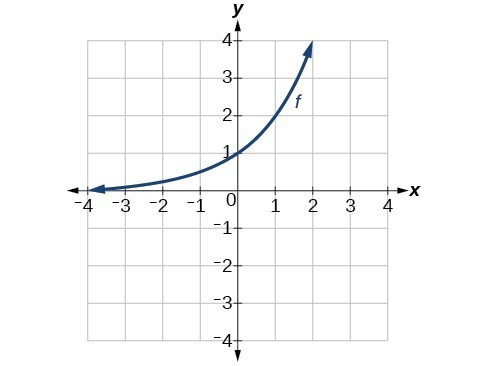24. $g\left(x\right)={2}^{x}+1$

25. $h\left(x\right)={2}^{x}-3$

26. $w\left(x\right)={2}^{x - 1}$

For the following exercises, sketch a graph of the function as a transformation of the graph of one of the toolkit functions.

27. $f\left(t\right)={\left(t+1\right)}^{2}-3$

28. $h\left(x\right)=|x - 1|+4$

29. $k\left(x\right)={\left(x - 2\right)}^{3}-1$

30. $m\left(t\right)=3+\sqrt{t+2}$

31. Tabular representations for the functions $f,g$, and $h$ are given below. Write $g\left(x\right)$ and $h\left(x\right)$ as transformations of $f\left(x\right)$.

 $x$ −2 −1 0 1 2 $f\left(x\right)$ −2 −1 −3 1 2
 $x$ −1 0 1 2 3 $g\left(x\right)$ −2 −1 −3 1 2
 $x$ −2 −1 0 1 2 $h\left(x\right)$ −1 0 −2 2 3

32. Tabular representations for the functions $f,g$, and $h$ are given below. Write $g\left(x\right)$ and $h\left(x\right)$ as transformations of $f\left(x\right)$.

 $x$ −2 −1 0 1 2 $f\left(x\right)$ −1 −3 4 2 1
 $x$ −3 −2 −1 0 1 $g\left(x\right)$ −1 −3 4 2 1
 $x$ −2 −1 0 1 2 $h\left(x\right)$ −2 −4 3 1 0

For the following exercises, write an equation for each graphed function by using transformations of the graphs of one of the toolkit functions.

33.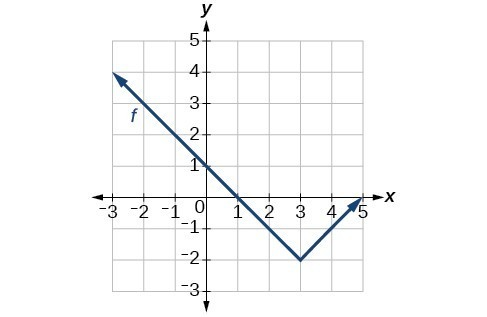34.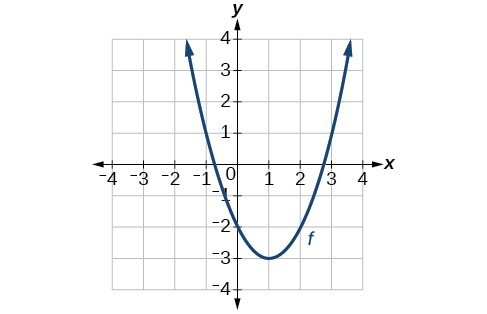35.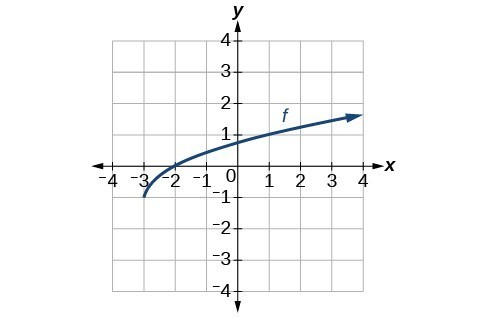36.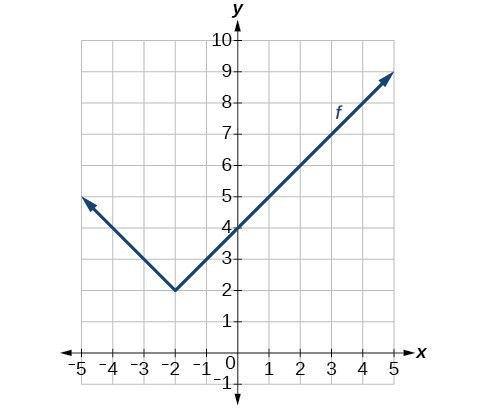37.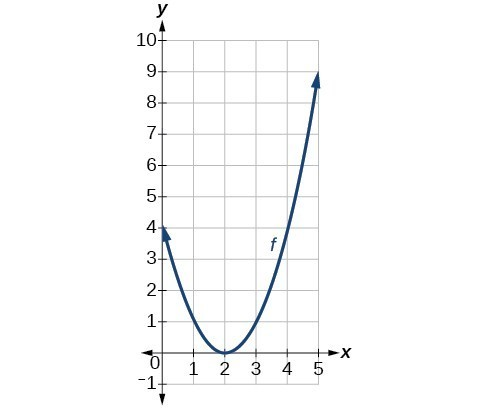38.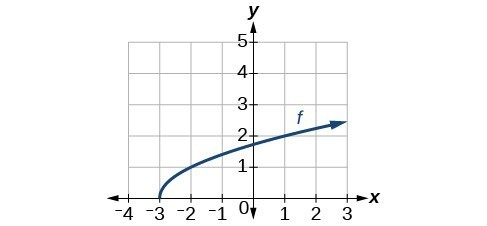39.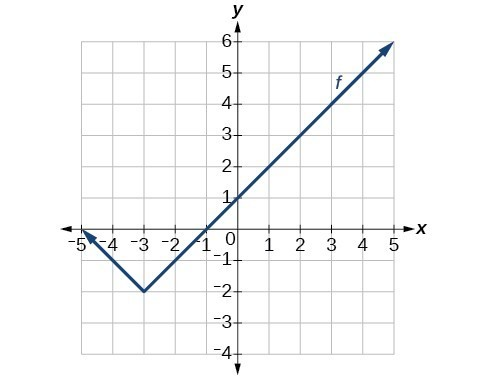40.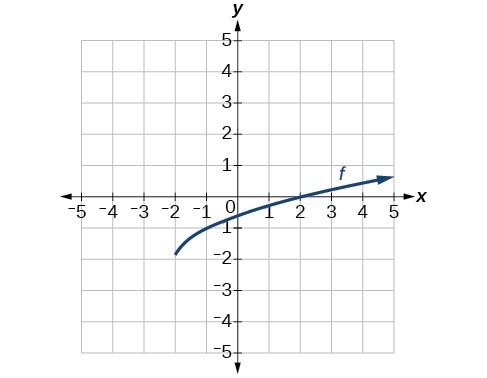For the following exercises, use the graphs of transformations of the square root function to find a formula for each of the functions.

41.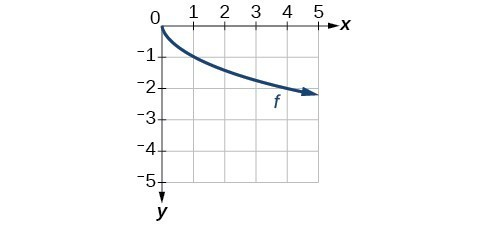42.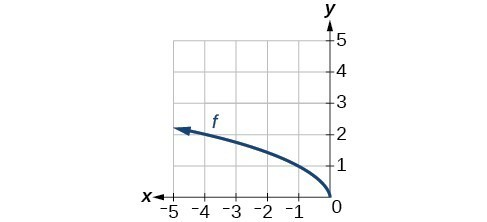For the following exercises, use the graphs of the transformed toolkit functions to write a formula for each of the resulting functions.

43.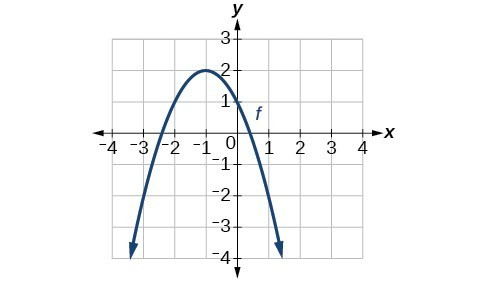44.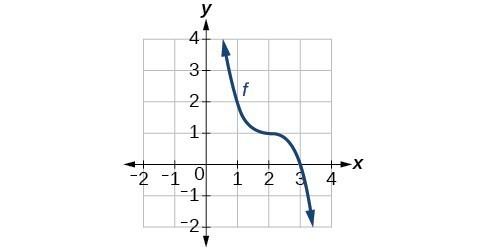45.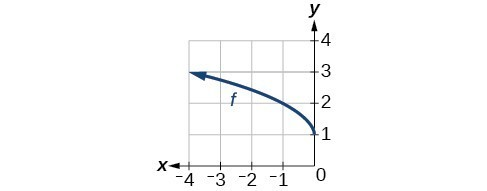46.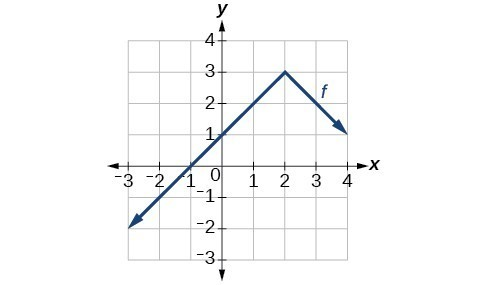For the following exercises, determine whether the function is odd, even, or neither.

47. $f\left(x\right)=3{x}^{4}$

48. $g\left(x\right)=\sqrt{x}$

49. $h\left(x\right)=\frac{1}{x}+3x$

50. $f\left(x\right)={\left(x - 2\right)}^{2}$

51. $g\left(x\right)=2{x}^{4}$

52. $h\left(x\right)=2x-{x}^{3}$

For the following exercises, describe how the graph of each function is a transformation of the graph of the original function $f$.

53. $g\left(x\right)=-f\left(x\right)$

54. $g\left(x\right)=f\left(-x\right)$

55. $g\left(x\right)=4f\left(x\right)$

56. $g\left(x\right)=6f\left(x\right)$

57. $g\left(x\right)=f\left(5x\right)$

58. $g\left(x\right)=f\left(2x\right)$

59. $g\left(x\right)=f\left(\frac{1}{3}x\right)$

60. $g\left(x\right)=f\left(\frac{1}{5}x\right)$

61. $g\left(x\right)=3f\left(-x\right)$

62. $g\left(x\right)=-f\left(3x\right)$

For the following exercises, write a formula for the function $g$ that results when the graph of a given toolkit function is transformed as described.

63. The graph of $f\left(x\right)=|x|$ is reflected over the $y$ axis and horizontally compressed by a factor of $\frac{1}{4}$ .

64. The graph of $f\left(x\right)=\sqrt{x}$ is reflected over the $x$ -axis and horizontally stretched by a factor of 2.

65. The graph of $f\left(x\right)=\frac{1}{{x}^{2}}$ is vertically compressed by a factor of $\frac{1}{3}$, then shifted to the left 2 units and down 3 units.

66. The graph of $f\left(x\right)=\frac{1}{x}$ is vertically stretched by a factor of 8, then shifted to the right 4 units and up 2 units.

67. The graph of $f\left(x\right)={x}^{2}$ is vertically compressed by a factor of $\frac{1}{2}$, then shifted to the right 5 units and up 1 unit.

68. The graph of $f\left(x\right)={x}^{2}$ is horizontally stretched by a factor of 3, then shifted to the left 4 units and down 3 units.

For the following exercises, describe how the formula is a transformation of a toolkit function. Then sketch a graph of the transformation.

69. $g\left(x\right)=4{\left(x+1\right)}^{2}-5$

70. $g\left(x\right)=5{\left(x+3\right)}^{2}-2$

71. $h\left(x\right)=-2|x - 4|+3$

72. $k\left(x\right)=-3\sqrt{x}-1$

73. $m\left(x\right)=\frac{1}{2}{x}^{3}$

74. $n\left(x\right)=\frac{1}{3}|x - 2|$

75. $p\left(x\right)={\left(\frac{1}{3}x\right)}^{3}-3$

76. $q\left(x\right)={\left(\frac{1}{4}x\right)}^{3}+1$

77. $a\left(x\right)=\sqrt{-x+4}$

For the following exercises, use the graph below to sketch the given transformations.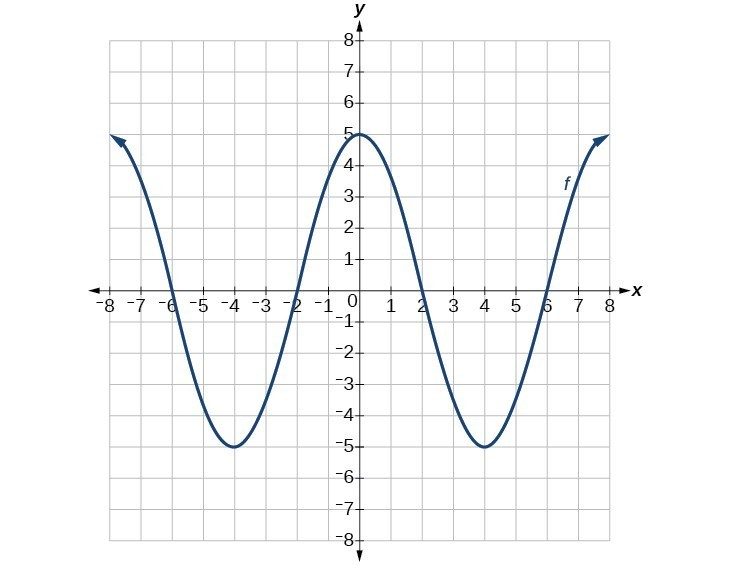78. $g\left(x\right)=f\left(x\right)-2$

79. $g\left(x\right)=-f\left(x\right)$

80. $g\left(x\right)=f\left(x+1\right)$

81. $g\left(x\right)=f\left(x - 2\right)$